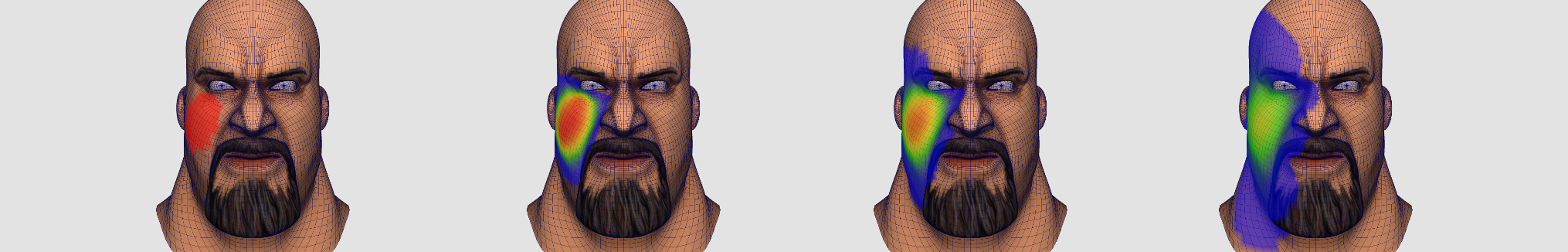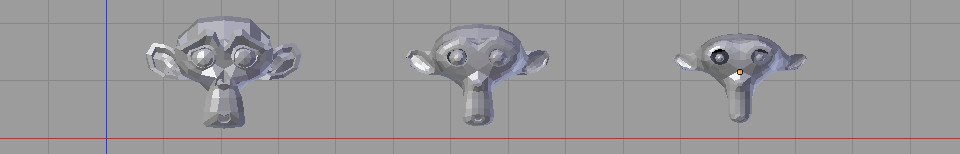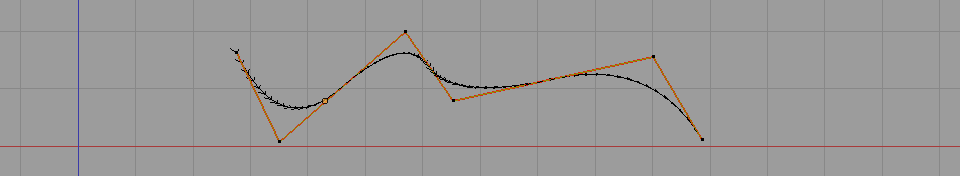# Find a tetrahedron circumcenter# Curvature of a parametric curve# Compute Bi-harmonic weights over a triangular mesh# Definition: Laplacian Matrix for triangle meshes# How to compute accurate vertex normals on irregular triangle meshes# Diffusing / smoothing weight map over a triangular mesh.Showcasing simple procedures with C++ code to smooth / diffuse per vertex weights over a triangle mesh.

# Laplacian smoothing (C++ code to smooth a mesh)Dropping a procedure to compute the Laplacian smoothing of a 3D mesh (with cotangent weights).

# C++ code for cotangent weights over a triangular mesh# 2D biharmonic stencil a.k.a bilaplacian operatorDraft / notes / memo

# Voro++.0.4.5 with cmake for easy compilation under windowsDropping my code of thecmake version of voro++0.4.5 ]. It helped me to compile the voro++ library under windows with cmake.

# Harmonic function: definitions and properties# How to generate bounded harmonic weights on a regular grid# Source code for poisson disk sampling of a triangle mesh# Curvature of a triangle mesh, definition and computation.Defining and giving the formula to compute the curvature over a triangle mesh at some vertices.

# C++ code for spline curves# Compute Harmonic weights on a triangular mesh• 1